# Loops in C++

## Loops in C++ with Examples:

Hey guys, welcome back to C++ basics, here in this article, I am going to discuss Loops in C++ with definitions, syntax, flow charts, and examples. Please read our last article, where we discussed Conditional Statements in C++ with Examples. In this article, we are going to discuss one of the core concepts of any programming called Loops. Loop Control Statements are very, very important for logical programming. If you are new to programming then please keep in mind that if you understand the working of the loop correctly you could solve most of the problems in the real world. So, keep a keen interest in this article while reading.

##### Loops in C++:

Loops are also called repeating statements or iterative statements. Loops play a very key role in programming. If you’re coming from mathematics and mathematics doesn’t have loops. So, here you have to learn something new in programming and this needs a lot of practice. The Looping Statements are also called Iteration Statements. So, we can use the word Looping and Iteration and meaning are the same.

##### What is looping?

The process of repeatedly executing a statement or group of statements until the condition is satisfied is called looping. In this case, when the condition becomes false the execution of the loops terminates. The way it repeats the execution of the statements will form a circle that’s why iteration statements are called loops.

Loop is nothing but repeating some tasks for some amount of time until the condition is true. There are two types of loops

1. Counter loops
2. Conditional loops

Before explaining, what are the counter and conditional loops let’s make you understand where we see loops in reality with real-time examples.

##### Loops exist in our daily routine.

Example: Every day I woke up at 6 ->go to jogging ->come home->take a bath->had breakfast-> went to college/office->work/learn->come back at 6-> watched tv/mobile->had dinner -> went to sleep this routine is repeated every day without change and this we call it has loops in programming.

Here even when you wake up tomorrow you do the same following thing and this continues until you are alive so here condition to break the loop is you have to die. Now let us explain what is the counter loop and conditional Loop.

1. Counter loops are loop, which executes a specific set of instructions for a certain number of times. Example: Token system followed in hospitals where the whole intension could be getting the headcount of patients.
2. Conditional Loops is executing a specific task until the condition is true. Example: Attend the online classes until the covid-19 situation comes to control.
##### Why do we need looping?

The basic purpose of the loop is code repetition. In implementation whenever the repetitions are required, then in place of writing the statements, again and again, we need to go for looping. Now let us see what are the different types of Loops available in C++.

The whole intention of using loops in programming is to make the developer’s job easy and make the code look clean and efficient. The developer goes for loops whenever he wants to execute some instructions a certain number of times. To give you a better understanding of the importance of loops in programming let us write a code without loops and with loops.

##### Program to print numbers from 1 to 10 without loops.

Till now what we learned using those concepts If I write a program to print 1 to 10 it looks something like this.

```#include <iostream>
using namespace std;
int n = 0;

int main() {
cout<<++n<<endl;

if (n >= 10) {
exit(0);
}
cout<<++n<<endl;

if (n >= 10) {
exit(0);
}
cout<<++n<<endl;

if (n >= 10) {
exit(0);
}
cout<<++n<<endl;

if (n >= 10) {
exit(0);
}
cout<<++n<<endl;

if (n >= 10) {
exit(0);
}
cout<<++n<<endl;

if (n >= 10) {
exit(0);
}
cout<<++n<<endl;

if (n >= 10) {
exit(0);
}
cout<<++n<<endl;

if (n >= 10) {
exit(0);
}
cout<<++n<<endl;

if (n >= 10) {
exit(0);
}
cout<<++n<<endl;

if (n >= 10) {
exit(0);
}

return 1;
}
```
###### Output: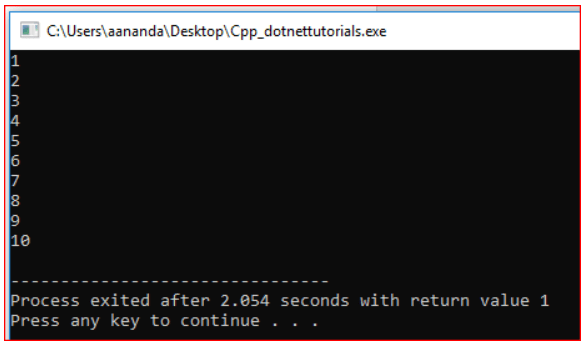Note: Even though we are able to print the numbers from 1 to 10, the code doesn’t look good as the same instruction is written multiple times also what is it if want to print from 1 to 1000? Or from 1 to 100000? So, without loops from not even looks understandable and efficient.

##### Program to print 1 to N with loop
```#include <iostream>
using namespace std;
int main ()
{
for (int n = 1; n <= 10; ++n)
{
cout << n << endl;
}
return 1;
}
```
###### Output: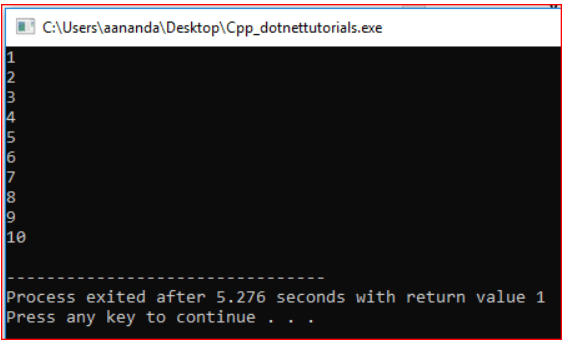Note: The above for loop is an example of a counter loop where the loop runs a specific number of times. Syntax and other things will be discussed soon.

The above code looks simple and readable. In addition, if I want to print from 1 to 1000 just, I need to change n<=10 to n<=1000 that’s it. So, code can be easily maintainable.

Program with loops takes more execution time compared to program without loops. In the above output screenshot, you could see a program without loops takes 2.054 seconds to print the value from 1 to 10 whereas a program with loops takes 5.276 to print from 1 to 10, and execution time is almost double when compared to a program without loops.

##### Types of Loops in C++

Iteration statements create loops in the program. It repeats the same code fragment several times until a specified condition is satisfied is called iteration. Iteration statements execute the same set of instructions until a termination condition is met. C++ provides the following loop for iteration statements: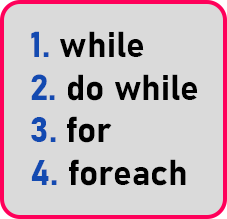In this article, we will give you an introduction to the loops, and also, we will be explaining these loops. Let’s first understand the flowchart.

##### Flowchart of Loop:

Let us understand the flowchart of the loop step by step for a better understanding.

###### Step 1:

This is the starting point of the flowchart.###### Step 2:

Here we are taking the input from the user, whatever the problem is, and some input it is taking.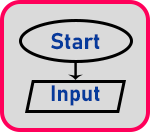###### Step 3:

It is processing the input.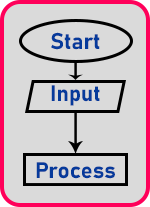###### Step 4: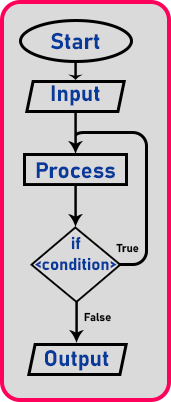As the input is processed then it checks for the condition, if the condition is true then again it goes back and processing will do and then again check for the condition, if the condition is true then again goes back, and so on.

This will be repeated. So, this processing part will go on repeating as long as that condition is true and once the conditions become false it will come out from here and print the output.

###### Step 5: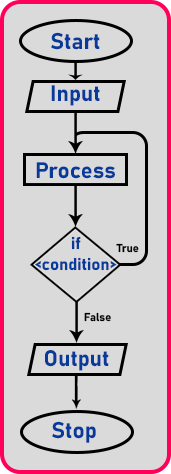Here our flowchart is finished. So, in any procedure, if we have to repeatedly execute some set of statements then we can repeatedly execute them using the loop. So, a loop is used for repeatedly executing some set of statements.

##### Realtime Example:

This type of thing we do commonly in our daily life repeatedly. We perform some steps like you have a coffee and you have to add some sugar in that. So, you’ll put some sugar and check it. If it’s not sweet yet then we will put some more sugar and check again. If it is sufficiently sweet then you will stop adding the sugar. So, this is a repeating procedure.

Let us take one more example. You are walking towards your home. If you have not reached home then take a step walk and then walk and check, have you reached home? No, then take one or more steps and check again. If yes, stop walking, and if no, you will be going on taking steps.

One more thing, we have seen in the number system like converting a decimal number to a binary number, we will divide that number by two and keep dividing until it becomes zero. We solve these problems which have repeating steps using these different loops:

1. while
2. do-while
3. for
4. for each